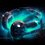# $\displaystyle \prod_{n=1}^{\infty} (1-x^n)^{24}$ coefficients

$P(x) = \displaystyle \prod_{n=1}^{\infty} (1-x^n)^{24}$

"Unwrapping" the product, you get a polynomial with coefficients $a_n \in \mathbb{R}$ :

$P(x) = a_0 + a_1 x + a_2 x^2 + ...$

Prove whether or not there exists a coefficient $a_n = 0$ in this polynomial. This is a problem I've been attempting to solve for over a week now but have made no significant progress on after turning the product (without the 24th power) into an infinite series. Could anyone offer some insight, please?Note by Arsenii Zharkov
1 year ago

This discussion board is a place to discuss our Daily Challenges and the math and science related to those challenges. Explanations are more than just a solution — they should explain the steps and thinking strategies that you used to obtain the solution. Comments should further the discussion of math and science.

When posting on Brilliant:

• Use the emojis to react to an explanation, whether you're congratulating a job well done , or just really confused .
• Ask specific questions about the challenge or the steps in somebody's explanation. Well-posed questions can add a lot to the discussion, but posting "I don't understand!" doesn't help anyone.
• Try to contribute something new to the discussion, whether it is an extension, generalization or other idea related to the challenge.

MarkdownAppears as
*italics* or _italics_ italics
**bold** or __bold__ bold
- bulleted- list
• bulleted
• list
1. numbered2. list
1. numbered
2. list
Note: you must add a full line of space before and after lists for them to show up correctly
paragraph 1paragraph 2

paragraph 1

paragraph 2

[example link](https://brilliant.org)example link
> This is a quote
This is a quote
    # I indented these lines
# 4 spaces, and now they show
# up as a code block.

print "hello world"
# I indented these lines
# 4 spaces, and now they show
# up as a code block.

print "hello world"
MathAppears as
Remember to wrap math in $$ ... $$ or $ ... $ to ensure proper formatting.
2 \times 3 $2 \times 3$
2^{34} $2^{34}$
a_{i-1} $a_{i-1}$
\frac{2}{3} $\frac{2}{3}$
\sqrt{2} $\sqrt{2}$
\sum_{i=1}^3 $\sum_{i=1}^3$
\sin \theta $\sin \theta$
\boxed{123} $\boxed{123}$

Sort by:

So far I think my most significant progress has been getting it into the form $\displaystyle \prod_{n=1}^{\infty} (1-x^n)^{24} = \sum_{n=-\infty}^{\infty} (-1)^n x^{\frac {3n^2-n}{2} }$

- 1 year ago

If the statement you said that$P(x)=a_0+a_1x+a_2 x^2+a_3 x^3+....$ then , $P(0)=a_0,(\frac{d}{dx}P(0)) =a_1 ,(\frac{d^2}{dx^2}\frac{P(0)}{2!}) =a_2,...$and so on. So if any $a_n$ to be zero the derivative of the product defined at zero must be zero.This is my insight and I hope you will get some idea , personal I feel it won't have a zero coefficient.

- 1 year ago

Here due to my bad knowledge of latex the derivative of the product has to be calculated first then put x=0.It's a pretty good question has many applications.

- 1 year ago

Plus the basic idea is using the Taylor series expantion,to denote the coefficient.

- 1 year ago

Unfortunately I haven't managed to find a good way to take the derivative of the function, but I did figure out the first 5 coefficients using combinatorics. For x, there's 24 "blocks" to choose from, For x^2, either 24 x^2's to choose from or 24 x's to choose 2 of from, etc., but I've been struggling with coming up with any form of general formula that actually confirms the absence of an $a_n=0$

- 1 year ago

- 1 year ago

- 1 year ago

- 1 year ago

- 1 year ago